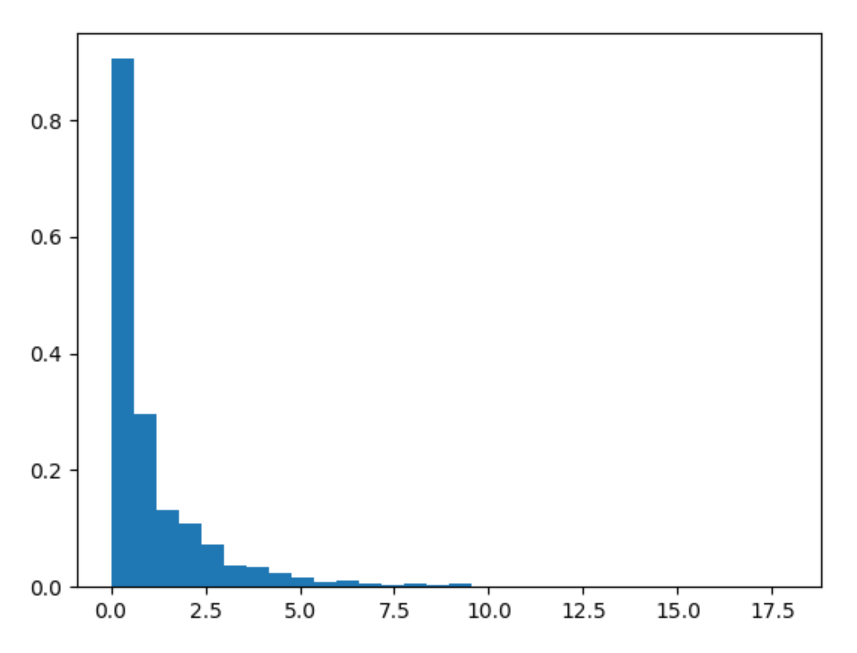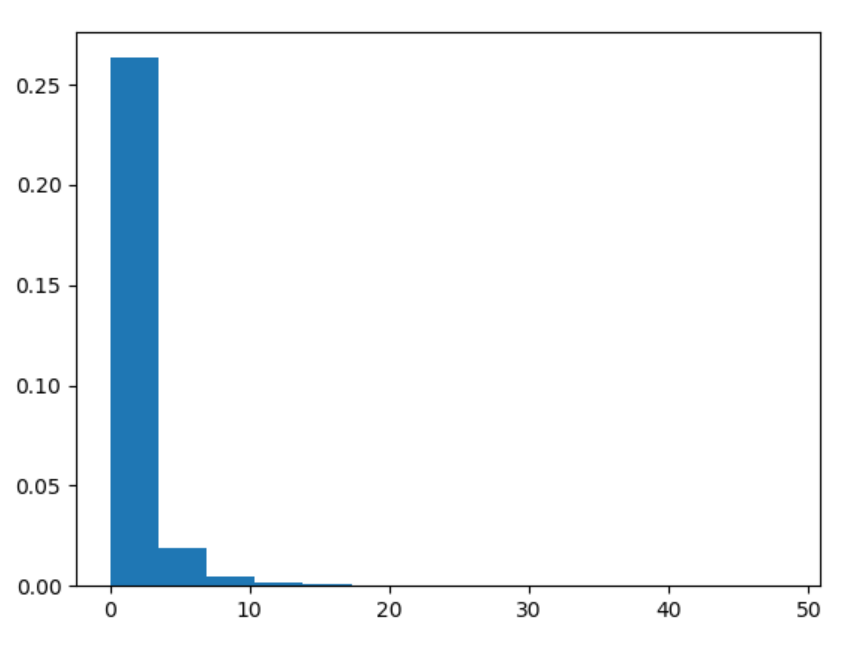# numpy.random.f() in Python

• Last Updated : 15 Jul, 2020

With the help of numpy.random.f() method, we can get the random samples of F distribution and return the random samples of numpy array by using this method.

Syntax : numpy.random.f(dfnum, dfden, size=None)

Attention geek! Strengthen your foundations with the Python Programming Foundation Course and learn the basics.

To begin with, your interview preparations Enhance your Data Structures concepts with the Python DS Course. And to begin with your Machine Learning Journey, join the Machine Learning - Basic Level Course

Return : Return the random samples as numpy array.

Example #1 :

In this example we can see that by using numpy.random.f() method, we are able to get the random samples of F distribution and return the random samples by using this method.

## Python3

 `# import numpy and f``import` `numpy as np``import` `matplotlib.pyplot as plt`` ` `# Using f() method``gfg ``=` `np.random.f(``0.98``, ``15.43``, ``1000``)`` ` `count, bins, ignored ``=` `plt.hist(gfg, ``30``, density ``=` `True``)``plt.show()`

Output :Example #2 :

## Python3

 `# import numpy and f``import` `numpy as np``import` `matplotlib.pyplot as plt`` ` `# Using f() method``gfg ``=` `np.random.f(``14.56``, ``31.45``, ``30000``)``gfg1 ``=` `np.random.f(gfg, ``10.45``, ``30000``)`` ` `count, bins, ignored ``=` `plt.hist(gfg1, ``14``, density ``=` `True``)``plt.show()`

Output :My Personal Notes arrow_drop_up